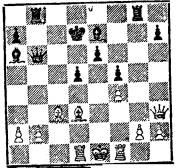Appendix to lecture 6-08-00

1. Below is the diagram that illustrates the combination discussed in the beginning of the lecture.1. The solution of the problem: the black starts

…….    L f8-f3

If ph2:Lf3     Q b6- e3

If Qh3: Lf3    be7-h4 and the black are loosing the Quen.

1. This is the formula elaborated by Claude Shannon to measure the value of a position

f(P)= 200(KK') + 9(QQ') + 5(RR') + 3(BB' + NN')_
.5(DD' + SS' + II') + .1(MM') + . . . ,

in which: -

K, Q, R, B, N, P is the number of White kings, queens, bishops, knights, and pawns on the board.

D, S, I are doubled, backward, and isolated White pawns.

M is  White’s mobility (measured, say, as the number of legal moves available to White).

Prime letters are the similar quantities for Black.

The coefficients 9, 5, and 3 are the widely accepted valuations of relevant chess pieces; the coefficient 200 for the king allows recognizing the end of the game. The coefficients .5 and .1 are merely Shannon’s rough estimates of corresponding positional parameters.